The Potentiometer-Internal resistance of a cell

# Objective

To measure the internal resistance of a given primary cell using potentiometer.

# Theory

## Potentiometer

Potentiometer is a device used to measure the internal resistance of a cell, to compare the e.m.f. of two cells and potential difference across a resistor. It consists of a long wire of uniform cross sectional area and of 10 m in length. The material of wire should have a high resistivity and low temperature coefficient.  The wires are stretched parallel to each other on a wooden board. The wires are joined in series by using thick copper strips. A metre scale is also attached on the wooden board.

It works on the principle that when a constant current flows through a wire of uniform cross sectional area, potential difference between its two points is directly proportional to the length of the wire between the two points.

Relation between e.m.f., potential difference, and internal resistance of a cell

If a cell of emf E and internal resistance r, connected to an external resistance R, then the circuit has the total resistance (R+r). The current I in the circuit is given by,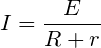or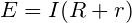Hence,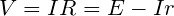This means, V is less than E by an amount equal to the fall of potential inside the cell due to its internal resistance.

From the above equation,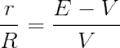Or;   The internal resistance of the cell,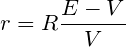Using a potentiometer, we can adjust the rheostat to obtain the balancing lengths l1 and l2 of the potentiometer for open and closed circuits respectively.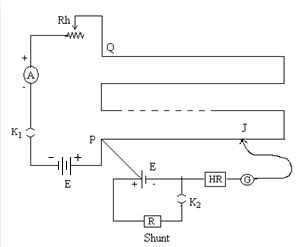Then, E= k l1   and   V = k l2  ; where k is the potential gradient along the wire.

Now we can modify the equation for getting the internal resistance of the given cell, by using the above relations as;# Learning Outcomes

• Students get the idea of potentiometer apparatus and its parts.
• Students are able to construct circuits based on circuit diagrams.
• Students understand the different component used in the experiment.

Cite this Simulator: